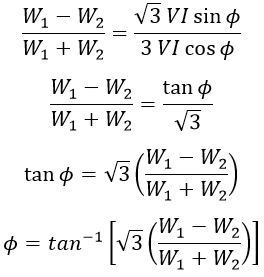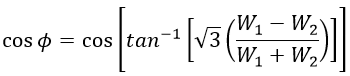# Three Phase Power Measurement Using One Wattmeter Method

A wattmeter is an instrument used to measure the electrical power supplied in a circuit. It consists of a voltage coil connected across the load and a current coil connected in series with the load. So that deflection of the pointer is proportional to the magnetic field produced by the two coils, which is nothing but proportional to power.

## One Wattmeter Method of Power Measurement :

This method is used only for a balanced load. When a 3-phase system is connected to a balanced 3-phase load, then the entire power consumption of the load can be determined by using a single wattmeter, whose arrangement is shown below.

The wattmeter current coil is connected in series with any one of the lines and hence carries the full line current. The voltage coil is connected in such a way that, one end to the line in which the current coil is placed, the other end is connected to the remaining two lines through an SPDT switch, and hence the line voltage will be impressed on it.

Here an SPDT switch is used to change the voltage coil connection between two phases. Hence, the sum of readings of the wattmeter with two different positions of the switch will give the total power consumed by the load. Let the phase voltages of the phases R, Y, and B be VR, VY, and VB respectively the phase/line currents of the phases R, Y, and B be IR, IY, and IB respectively.

### Measurement of Power Factor Using One Wattmeter Method :

#### By subtracting W1 wth W2, we get,Dividing equations 2 and 1, we get,Therefore power factor,Power measurement in a 3-phase system using one wattmeter can be done only when the load is balanced. Any slight change in load on any phase produces a significant error in the readings. However, in order to measure 3-phase power in unbalanced load, two wattmeter or three wattmeter method is used.

Do not enter any spam links and messages